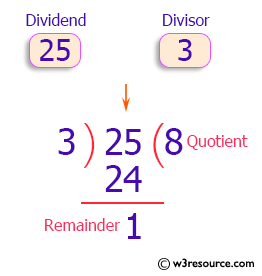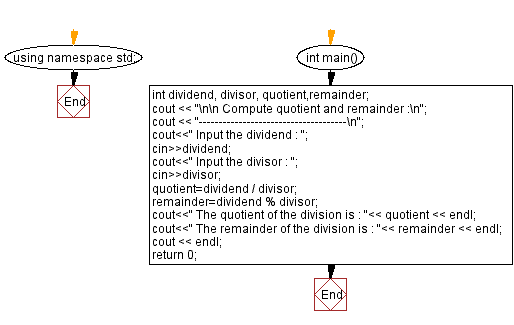﻿ C++ Exercises: Compute quotient and remainder - w3resource

# C++ Exercises: Compute quotient and remainder

## C++ Basic: Exercise-29 with Solution

Write a C++ program to compute the quotient and remainder.

Pictorial Presentation:Sample Solution:

C++ Code :

``````#include <iostream>
using namespace std;

int main()
{
int dividend, divisor, quotient,remainder;
cout << "\n\n Compute quotient and remainder :\n";
cout << "-------------------------------------\n";
cout<<" Input the dividend : ";
cin>>dividend;
cout<<" Input the divisor : ";
cin>>divisor;
quotient=dividend / divisor;
remainder=dividend % divisor;
cout<<" The quotient of the division is : "<< quotient << endl;
cout<<" The remainder of the division is : "<< remainder << endl;
cout << endl;
return 0;
}
``````

Sample Output:

``` Compute quotient and remainder :
-------------------------------------
Input the dividend : 25
Input the divisor : 3
The quotient of the division is : 8
The remainder of the division is : 1
```

Flowchart:C++ Code Editor:

What is the difficulty level of this exercise?

﻿

## C++ Programming: Tips of the Day

Why is there no std::stou?

The most pat answer would be that the C library has no corresponding "strtou", and the C++11 string functions are all just thinly veiled wrappers around the C library functions: The std::sto* functions mirror strto*, and the std::to_string functions use sprintf.

Ref: https://bit.ly/3wtz2qA

We are closing our Disqus commenting system for some maintenanace issues. You may write to us at reach[at]yahoo[dot]com or visit us at Facebook